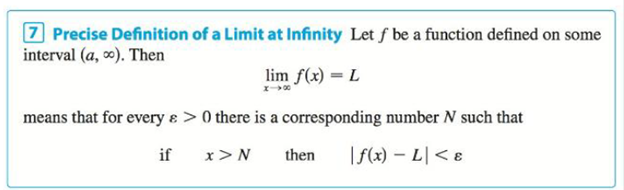Chapter 2.6, Problem 76E### Single Variable Calculus: Early Tr...

8th Edition
James Stewart
ISBN: 9781305270343

#### Solutions

Chapter
Section### Single Variable Calculus: Early Tr...

8th Edition
James Stewart
ISBN: 9781305270343
Textbook Problem

# (a) How large do we have to take x so that 1 x < 0.0001 ? (b) Taking r = 1 2 in Theorem 5, we have the statement lim x → ∞ 1 x = 0 Prove this directly using Definition 7.Definition 7(a)

To determine

To find: The large values of x when 1x<0.0001.

Explanation

Calculation:

Consider the inequality 1x<0.0001.

1x<0.00011x<110000

Take the reciprocal on both sides resulting by change in sign.

x>10000

Squaring on both sides

(b)

To determine

To prove: The limit function limx1x=0.

### Still sussing out bartleby?

Check out a sample textbook solution.

See a sample solution

#### The Solution to Your Study Problems

Bartleby provides explanations to thousands of textbook problems written by our experts, many with advanced degrees!

Get Started

#### In Exercises 516, evaluate the given quantity. log4164

Finite Mathematics and Applied Calculus (MindTap Course List)

#### In Exercises 73-80, find the indicated limits, if they exist. 79. limxx5x3+x1x6+2x2+1

Applied Calculus for the Managerial, Life, and Social Sciences: A Brief Approach

#### Given that for all x, a power series for is:

Study Guide for Stewart's Multivariable Calculus, 8th